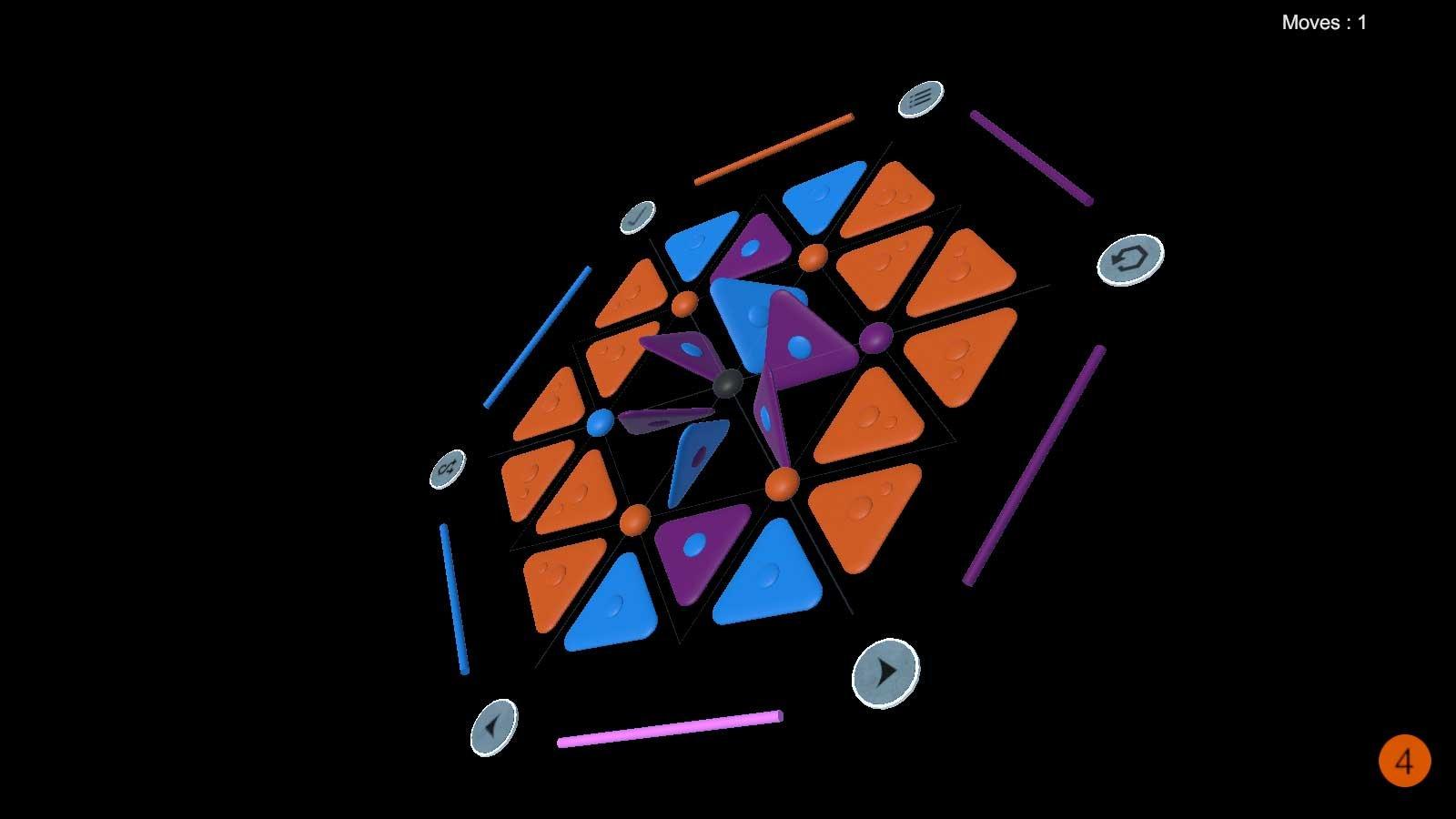## Primary LayoutA Trinagon puzzle consists of clearly defined elements :

• Triangles (here 24), equally distributet on the playground in a symmetrical pattern (here hexagonal).
• Rotators (here 7), sitting in the symetry centers of adjacent triangles. They are the spheres / smarties. Here every rotator has 6 triangles.

The Triangles have a

• Color ... one out of 10 possible colors

and can also have a

• Face ... up or down, marked with a colored dot.
• Direction ... one corner that is marked with a smaller dot.

The triangles can be moved around unsing the rotators. Each rotator moves its triangles around itself clockwise or counterclockwise, so that they are interchanged (permuted).

It's up to you to find the right moves so that the triangles create the right patterns.

There are 2 basic puzzle setups. One is simply shuffling a pattern and then finding back to the beginning (randomized puzzle), and the other is to go from a beginning pattern to a different solution pattern.